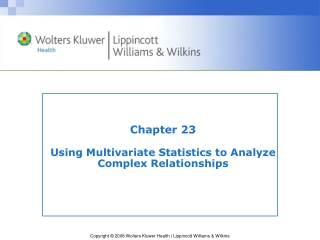DownloadDownload PresentationChapter 23 Using Multivariate Statistics to Analyze Complex Relationships

# Chapter 23 Using Multivariate Statistics to Analyze Complex Relationships

Télécharger la présentation## Chapter 23 Using Multivariate Statistics to Analyze Complex Relationships

- - - - - - - - - - - - - - - - - - - - - - - - - - - E N D - - - - - - - - - - - - - - - - - - - - - - - - - - -
##### Presentation Transcript

1. Multivariate Statistics • Statistical procedures for analyzing relationships among 3 or more variables • Most commonly used procedures: • Multiple regression • Analysis of covariance • Multivariate analysis of variance • Factor analysis • Logistic regression

2. Simple Linear Regression • Makes predictions about the values of one variable based on values of a second variable • Estimates a straight-line fit to the data that minimizes deviations from the line

3. Basic Linear Regression Equation Y= a + bX Where Y= predicted value of variable Y(dependent variable)a = intercept constantb = regression coefficient (slope of the line)X = actual value of variable X (independent variable) This equation solves for a and b such that sums of squares of prediction errors are minimized (least squares criterion)

4. Example of Simple Linear Regression

5. Multiple Linear Regression • Used to predict a dependent variable based on two or more independent (predictor) variables • Dependent variable is continuous (interval or ratio-level data) • Predictor variables are continuous or dichotomous (dummy variables)

6. Equation for Multiple Regression with Two Predictor Variables Y= a + b1X1 + b2X2 Where Y= predicted value of variable Y(dependent variable) a = intercept constant b1 = regression coefficient for variable X1 X1 = actual value of variable X1 b2 = regression coefficient for variable X2 X2 = actual value of variable X2

7. Multiple Correlation Coefficient (R) • Is the correlation index for a dependent variable and two or more independent variables • Does not have negative values: it shows strength of relationships, but not direction

8. Multiple Correlation Coefficient (R) (cont’d) • Can be squared (R2) to estimate the proportion of variability in the dependent variable accounted for by the independent variables • Cannot be less than the highest bivariate correlation between the dependent variable and an independent variable

9. Correlation Matrix

10. Strategies for Handling Predictors in Multiple Regression Simultaneous multiple regression Enters all predictor variables into the regression equation at the same time

11. Strategies for Handling Predictors in Multiple Regression (cont’d) Hierarchical multiple regression Enters predictors into the equation in a series of steps, controlled by researcher

12. Strategies for Handling Predictors in Multiple Regression (cont’d) Stepwise multiple regression Enters predictors in a series of empirically-determined steps, in the order that produces the greatest increment to R2

13. Beta Weights (s) • Are standardized regression coefficients (all in same metric) • Are sometimes used to estimate the relative importance of independent variables in the regression equation • Are used with standard scores (zX) rather than raw scores (X) • Standard scores transform raw scores to have a mean = 0 and an SD = 1

14. Analysis of Covariance (ANCOVA) Extends ANOVA by removing the effect of extraneous variables (covariates) before testing whether mean group differences are statistically significant

15. Analysis of Covariance (cont’d) Levels of measurement of variables: • Dependent variable is continuous • Independent variable is categorical (group) • Covariates are continuous or dichotomous

16. Fictitious Data for ANCOVA Example

17. MANOVA • Multivariate analysis of variance • Extension of ANOVA to more than one dependent variable • Used to test the significance of differences in group means for multiple dependent variables, considered simultaneously • Can be used with covariates: Multivariate analysis of covariance (MANCOVA)

18. Discriminant Analysis • Used to predict categorical dependent variables (e.g., compliant/noncompliant) based on two or more predictor variables • Accommodates predictors that are continuous or dichotomous • Produces an index indicating the proportion of variance in the dependent variable unaccounted for by predictor variables (Wilks’ lambda—)

19. Canonical Correlation • Analyzes the relationship between two or more independent variables and two or more dependent variables • Relationships are expressed by the canonical correlation coefficient (RC)

20. Causal Modeling • Tests a hypothesized multivariable causal explanation of a phenomenon • Includes: • Path analysis • Linear structural relations analysis (LISREL)

21. Path Analysis • Relies on multiple regression • Is applied to a prespecified model based on prior knowledge and theory • Tests recursive models—ones in which causation is assumed to be unidirectional • Results are often displayed in a path diagram

22. Example of a Path Diagram

23. Path Analysis (cont’d) • Distinguishes two types of variable: • Exogenous variable • Endogenous variable • Yields path coefficients—weights representing the effect of one variable on another; indicates relative importance of predictors

24. Structural Equations Modeling (SEM) • Another approach to causal modeling • Not as many assumptions and restrictions as path analysis • Can accommodate measurement errors, nonrecursive models that allow for reciprocal causal paths, and correlated errors

25. Phases of SEM • Measurement model phase • Structural equations modeling phase